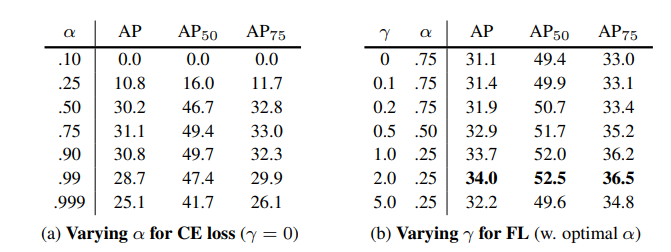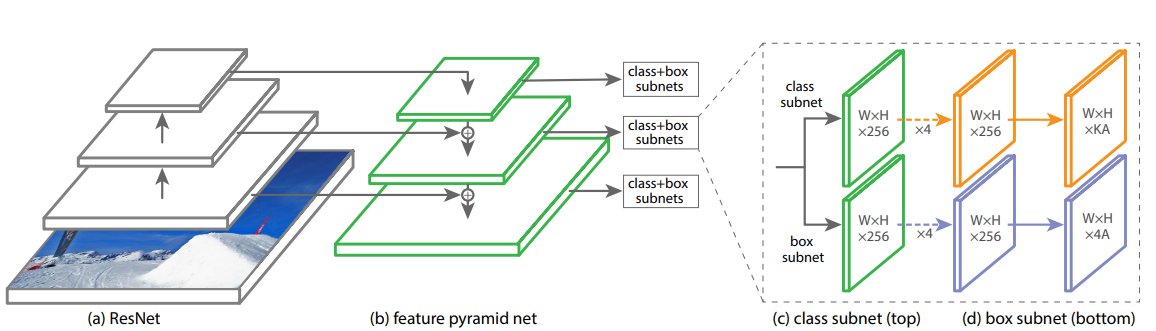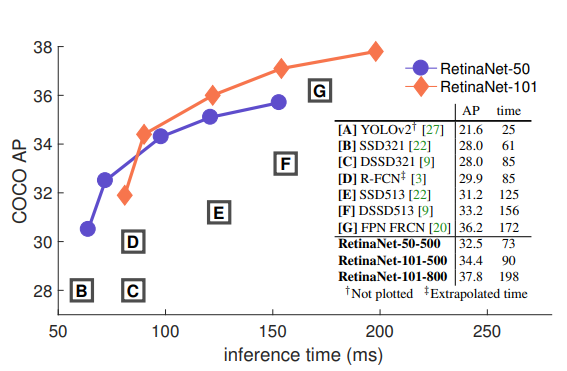# 目标检测(object detection)系列（十一） RetinaNet：one-stage检测器巅峰之作# RetinaNet原理

## 设计理念

two-stage方法会分两步完成目标检测，首先生产区域建议框，然后在对框做分类判别和回归矫正，而one-stage方法只有一步就完成了分类判别和bbox的回归。也就是因为这个不同，造成了上面的特点，首先耗时很好理解，因为two-stage有两步，而第二步的子网络要多次的重复输出，所以它不可避免的慢。那么造成two-stage效果好，one-stage效果偏差的本质原因是什么呢？

• FPN会选择与Ground turth的IOU>0.7的做正样本，与Ground turth的IOU<0.3的做负样本，这拉大正负样本间的差异；
• FPN的RPN最后的输出会控制在1000-2000个之间，控制样本数量；
• FPN组合每一次用于训练的minibatch，正负样本比例为1:3。

## Focal loss

Focal Loss是一种改进的交叉熵损失，一般情况下交叉熵损失在二分类时长这样：

C E ( p , y ) = − [ y l o g ( p ) + ( 1 − y ) l o g ( 1 − p ) ] CE(p,y)=-[ylog(p)+(1-y)log(1-p)]

C E ( p , y ) = { − l o g ( p ) i f ( y = 1 ) − l o g ( 1 − p ) o t h e r w i s e CE(p,y)=\left\{\begin{matrix} -log(p) &if (y=1) \\ -log(1-p) & otherwise \end{matrix}\right.

p t = { p i f ( y = 1 ) 1 − p o t h e r w i s e p_{t}=\left\{\begin{matrix} p &if (y=1) \\ 1-p & otherwise \end{matrix}\right.

α t = { α i f ( y = 1 ) 1 − α o t h e r w i s e \alpha_{t}=\left\{\begin{matrix} \alpha &if (y=1) \\ 1-\alpha & otherwise \end{matrix}\right.

C E ( p t ) = − α t l o g ( p t ) CE(p_{t})=-\alpha_{t}log(p_{t})

C E ( p , y ) = − { α l o g ( p ) i f ( y = 1 ) ( 1 − α ) l o g ( 1 − p ) o t h e r w i s e CE(p,y)=-\left\{\begin{matrix} \alpha log(p) &if (y=1) \\ (1-\alpha)log(1-p) & otherwise \end{matrix}\right.

F L ( p t ) = ( 1 − p t ) γ l o g ( p t ) FL(p_{t})=(1-p_{t})^{\gamma }log(p_{t})

F L ( p , y ) = − { ( 1 − p ) l o g ( p ) i f ( y = 1 ) p l o g ( 1 − p ) o t h e r w i s e FL(p,y)=-\left\{\begin{matrix} (1-p) log(p) &if (y=1) \\ p log(1-p) & otherwise \end{matrix}\right.

• 对于一个正例，模型认为它简单，那么 p p 会趋近于1， 1 − p 1-p 会趋近于0，损失就会变小，相反的就会变大。
• 对于一个负例，模型认为它简单，那么 p p 会趋近于0，损失就会变小，相反的就会变大。

F L ( p t ) = α t ( 1 − p t ) γ l o g ( p t ) FL(p_{t})=\alpha_{t}(1-p_{t})^{\gamma }log(p_{t})Focal loss是RetinaNet最重要的部分，网络结构、Anchor、损失等其余的东西RetinaNet用的都是之前，我们简单提一下吧。

## 网络结构## Anchor Box

RetinaNet选取Anchor Box的策略和FPN相似，一共有5个不同尺度的特征图，分别是 3 2 2 − 51 2 2 32^{2}-512^{2} ，每一层会有三种比例，所以FPN有15种Anchor，但是RetinaNet在这个基础上又加了一个因素，就是每一层特征图上还有一个尺度，它分别是该层特征图尺度的 2 0 , 2 1 3 , 2 2 3 {2^{0},2^{\frac{1}{3}},2^{\frac{2}{3}}} ，于是RetinaNet的Anchor变成了45个。因为Focal loss的引入，让Anchor的选取变得为所欲为，就是不怕多。╮(￣▽ ￣)╭

# RetinaNet性能评价04-24
09-277840
08-211万+
09-15
03-082204
©️2020 CSDN 皮肤主题: Age of Ai 设计师:meimeiellie